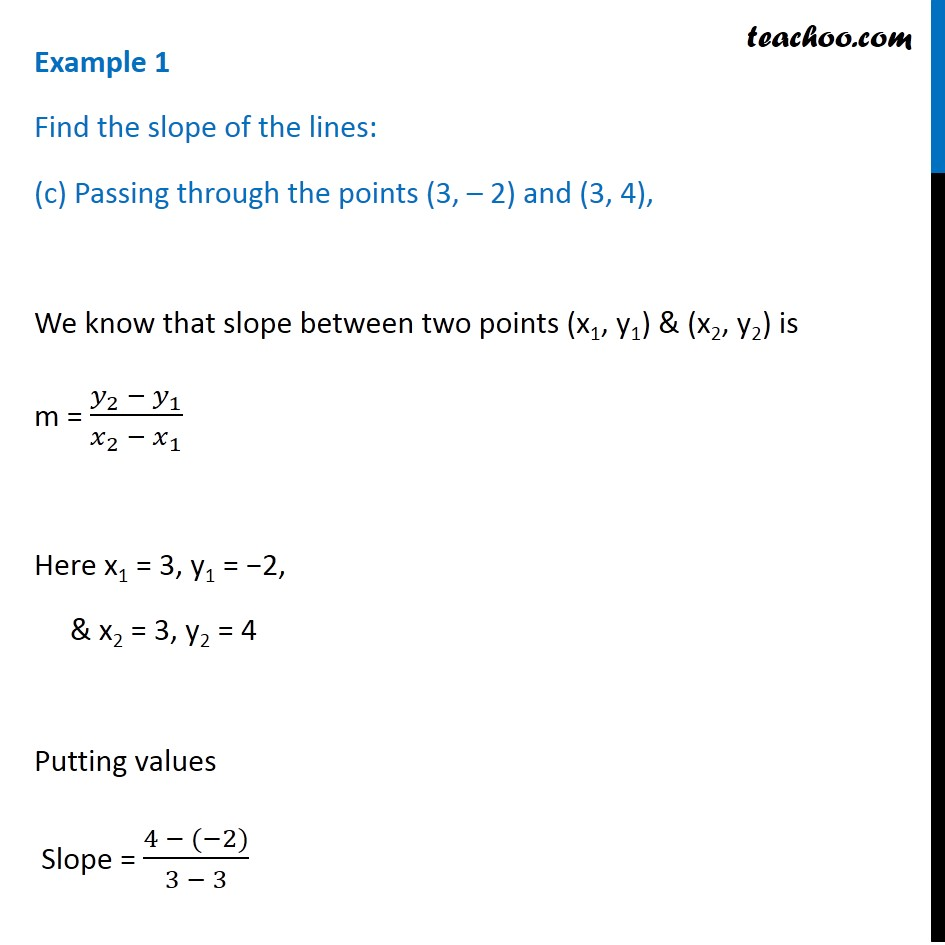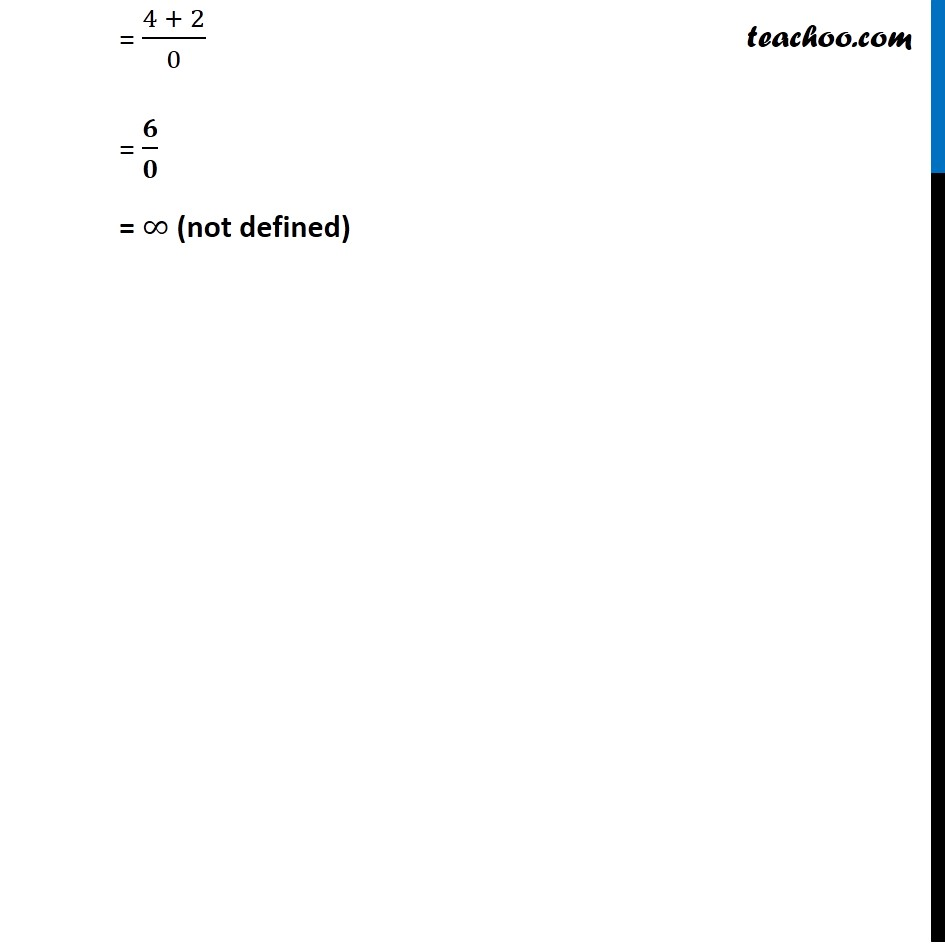Examples

Chapter 9 Class 11 Straight Lines
Serial order wiseLearn in your speed, with individual attention - Teachoo Maths 1-on-1 Class

### Transcript

Example 1 Find the slope of the lines: (c) Passing through the points (3, – 2) and (3, 4), We know that slope between two points (x1, y1) & (x2, y2) is m = (𝑦_2 − 𝑦_1)/(𝑥_2 − 𝑥_1 ) Here x1 = 3, y1 = −2, & x2 = 3, y2 = 4 Putting values Slope = (4 − (−2))/(3 − 3) = (4 + 2)/0 = 𝟔/𝟎 = ∞ (not defined)